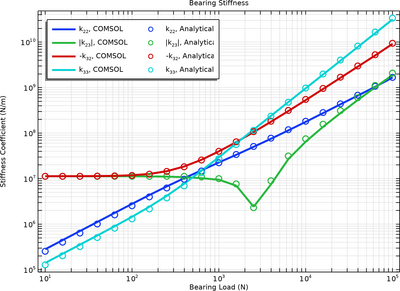# Application Gallery

#### Evaluation of Dynamic Coefficients of a Plain Journal Bearing

Application ID: 78201

When analyzing rotors, it is common that bearings are modeled through their effective dynamic coefficients about a static equilibrium position. This model illustrates how to compute such coefficients for a plain journal bearing. The bearing length is kept much smaller than its diameter in order to verify that the calculated coefficients match corresponding analytical values for a short bearing approximation.This model example illustrates applications of this type that would nominally be built using the following products: